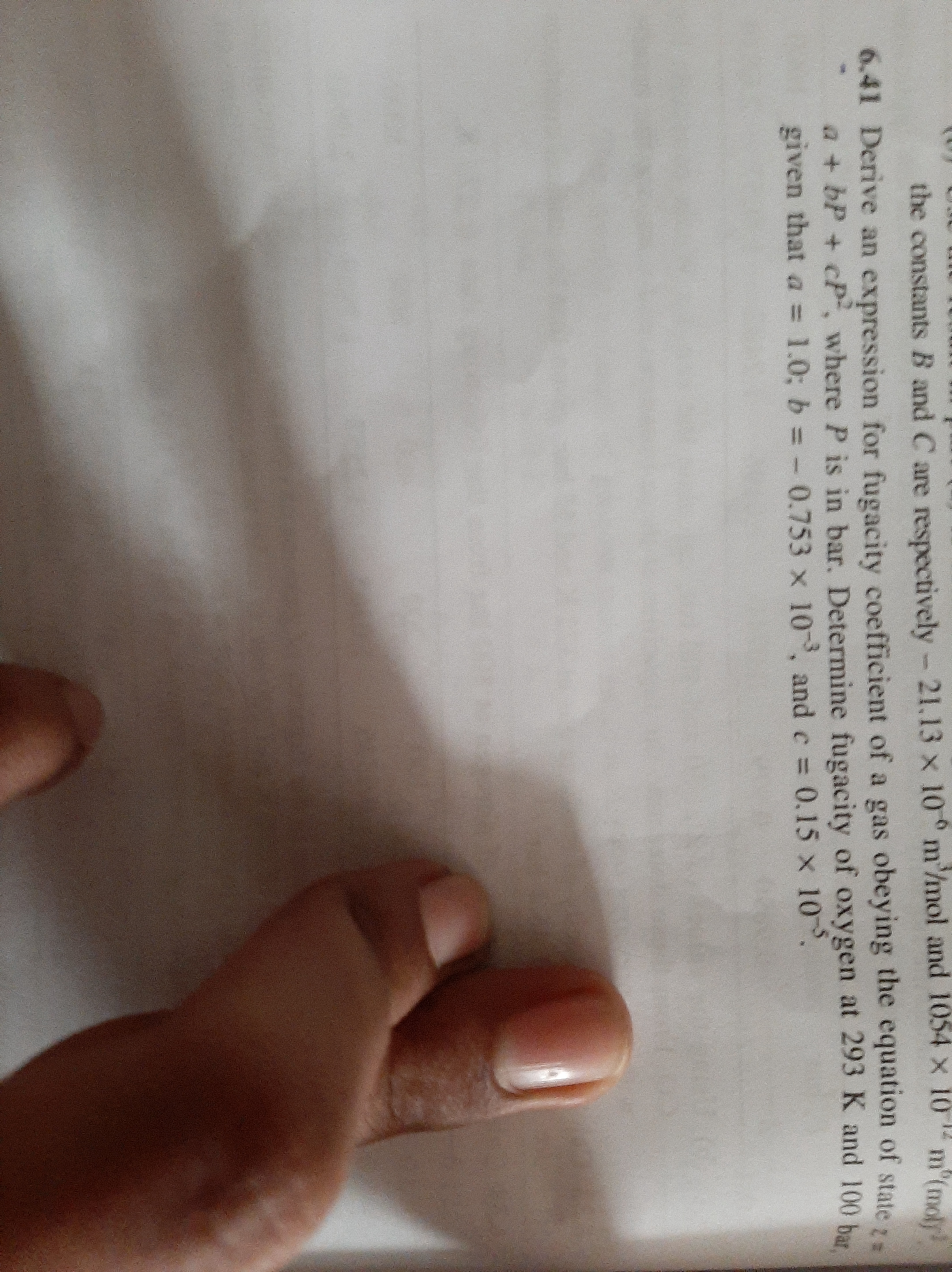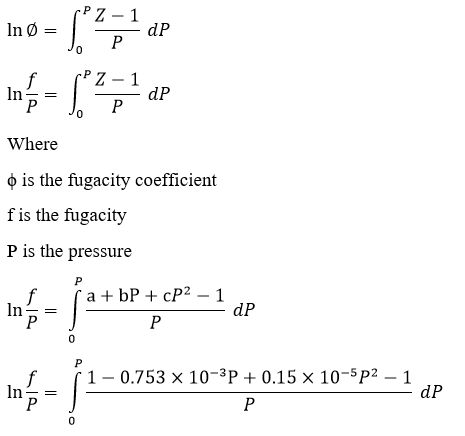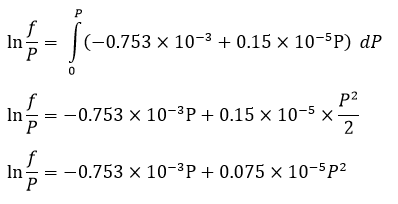# Derive an expression for fugacity coefficient of a gas obeying the equation

Question-AnswerCategory: Fluid MechanicsDerive an expression for fugacity coefficient of a gas obeying the equation

unsolved problem 6.41 on page no.272Derive an expression for fugacity coefficient of a gas obeying the equationStep 1Step 2Step 3